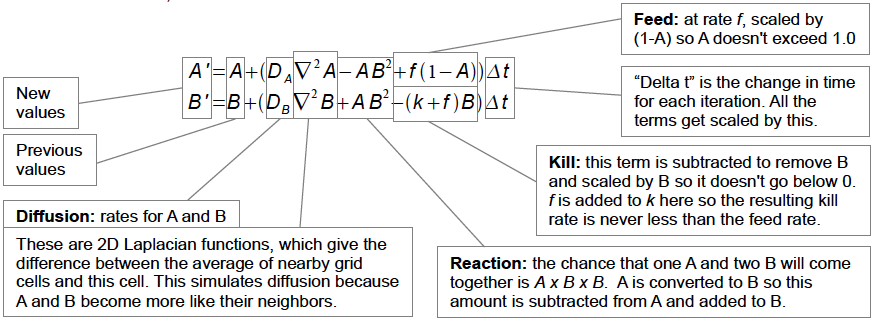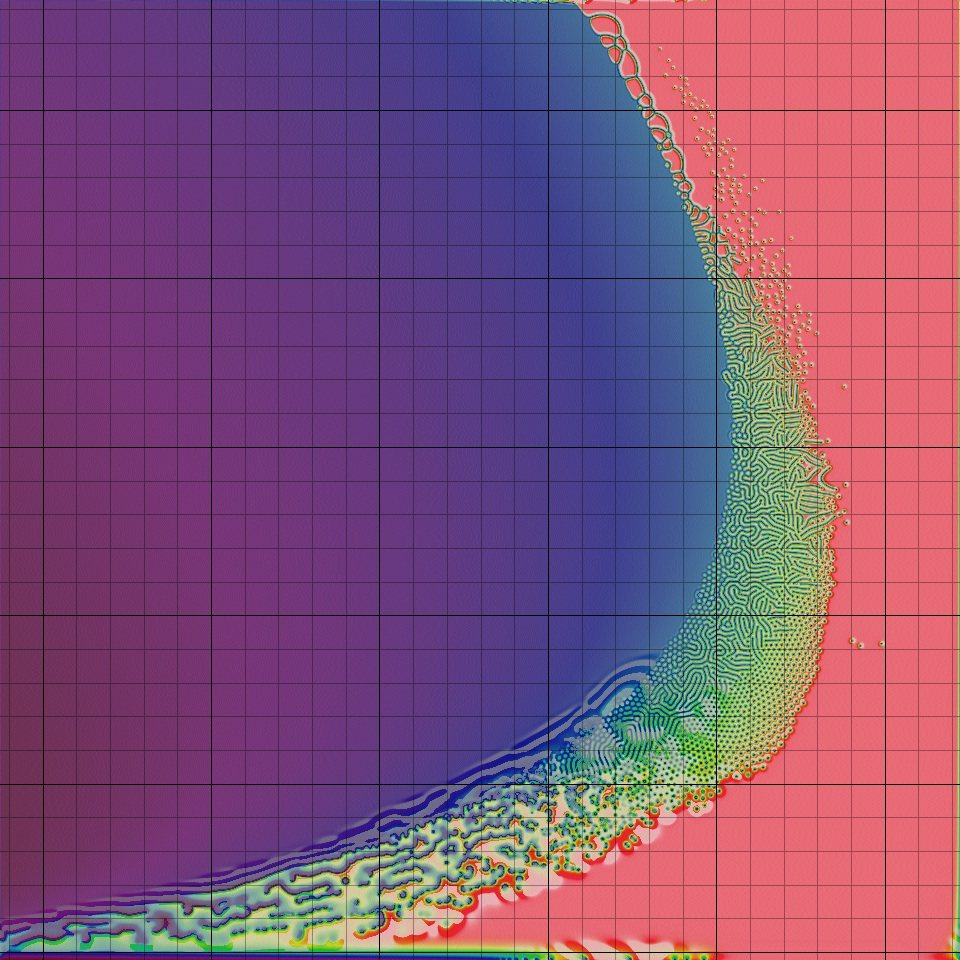# The beauty of computing patterns

I’ve always been enchanted by shapes and figures found in nature. From trees, leaves, animal’s coats or our own fingertips. As a math-and-code lover, I ended up reading Turing’s article on patterns in nature.

In 1952, he presented The chemical basis of Morphogenesis; where he introduced a system of equations to model changes in space and time from which certain patterns will be yield.

These are known as Reaction diffusion systems, and they are mathematical models which study the behavior of different phenomenons, such as the variation of concentration of one or more chemical substances.

And because I believe that the difference between words and code is like the difference between telling and doing. Let’s rather implement these models,and create some patterns with the power of computability!

### Reducing the system to one substance:

When throwing ink into water, the ink moves from higher concentrations to lower. This proccess is called diffusion, it is normally thought of as a smooth and stable process, and can be mathematically modelled with the following equation:Understanding formula by implementing it

What we need is defining a concentration value for each point in space, which will be changing while time goes by:

`C(x, t)`

Lets imagine time is discrete: t in [0 1 2 3 4 5 6 7 …]

For each moment t, for each x in space: we are defining C, the concentration of ink, according to a constant that represents the rate of diffusion [D]; and a value obtained from how the concentration in x changed according to its neighbourhood in the previous step [ N(x, t-1)]. For example, by taking the difference of the value of x and the average of its neighbourhood at t-1.

So our discrete interpretation of the formula would look something like this:

`C(x, t) = D * N(x, t-1)`

### Three.js + WebGL implementation

For creating a visualization of this system, it is important to notice that previous state will be needed for defining actual values. In other words, we will be using the output of previous step, for feeding the input of the actual one. These are known as feedback systems, and can be thought it as a sequence of frames:Each white square will be showing the color values at each position of the screen, and black arrows are the algorithm for updating those colors at each frame.

Specifically in diffusion, each pixel will be map to a color value representing the concentration of such color.

I used Three.js for rendering each frame, and WebGL for implementing the diffusion algorithm.

I implemented the Ping-pong rendering technique for being able to define actual frame in terms of last one. As we need somehow to save the information of previous frame.

Defining Three.js script general structure:

• Setting up the scenes
``````      var camera,renderer,scene;
function setupMainScene(){
...
}

var bufferScene ,ping ,pong, renderTargetParams;
function setupBufferScene(){
...

//initialize textures ping and pong
}
``````
• Initializing scenes
``````  var bufferUniforms, bufferMaterial, plane, bufferObject;
function initBufferScene(){
bufferUniforms = {
...
};

});

plane = new THREE.PlaneBufferGeometry( 2, 2 );
bufferObject = new THREE.Mesh( plane, bufferMaterial );
}

function initMainScene(){
finalMaterial =  new THREE.MeshBasicMaterial({map: ping});
[*]
quad = new THREE.Mesh( plane, finalMaterial );
}
``````
• Rendering the scene
``````  function render(){
requestAnimationFrame( render );
//Ping to buffer scene
renderer.setRenderTarget(ping);
renderer.render(bufferScene, camera);

renderer.setRenderTarget(null);
renderer.clear();

//Swap ping and pong
let temp = pong;
pong = ping;
ping = temp;

//Update uniforms
...

//Draw to screen
renderer.render( scene, camera );
}
``````

### Understanding how ping pong buffers workedWhere:

• STEP 0: Set up 2 scenes, main one and buffered one. Initialize 2 textures : ping and pong. One for each respective scene.
• STEP 1: Set texture ping to be rendered in the buffer scene.
• STEP 2-3-4: Swap textures.
• STEP 5: Update scene materials.

### Experimenting diffusion system

Code is available here## Going deep into reaction-diffusion systemsWe already have a sense of what diffusion is. Now let’s suppose that the system has more than one substance, which will be diffusing at different rates and reacting together.

Going back to Turing’s article. He described these systems, with two substances and called them morphogens.

In the reaction, morphogens works opposite to each other, as it is known in a growth-decay or activator-inhibtor relationship. And if as a pre-condition the reaction rate of the decay is faster the the growth rate, you would expect the system to behave smoothly and stable like a diffusion one, but here is where Turing introduced an amazing idea: known as “diffusion-driven instability”, where the diffusion rates of substances would carry an asymetry thorughout the solution, so under certain parameters these instabilities cause the formation of patterned concentrations.

For learning more I suggest reading pattern formation in the animal skin.

### Using Gray Scott model

It is a simulation of reaction-diffusion system of 2 virtual chemicals in a 2D grid. This tutorial of Karl Sims explains how the algorithm works. He describes the simulation in terms of the following equations:Making a analogy to our diffusion system interpretation, let’s imagine time is discrete [0 1 2 3 4 5 6 7 …].

Essentialy, for each cell(x,y) in your grid you have A concentration of one chemical, and B concentration of the other. And you are updating the values according to: it previous value, how it diffuse and how they react.

Reaction depends on 2 constant value: feed and kill rate, it is important to notice that only a special combinations of these values evolves in a pattern.

This is a great resource to explore those values.

You will see this a grid as these:Where the horizontal axis are the kill values, and the vertical the feed ones. By clicking over {kill, feed} cell, you will see how the system will evolve. The green-ish area, are the rates that we eventually would like to explore in our simulation.

Or can try values with Pearson’s classification

### Three.js + WebGL implementation

It uses the same ping pong technique descripted before for rendering frames, with the difference that at each frame there can be several iterations of the algorithm (arrows going down).[*] for Gray Scott model I changed form MeshBasicMaterial to Shader Material since I added an extra color shader.

# Gray Scott model:

Code is available here. For rendering just patterns, interpolation value should be 0.# Mapped on 3D-Surfaces:

Added a vertex shader for meshing to geometry. Experimental code is found in here# Gray Scott model mixed with video capture: